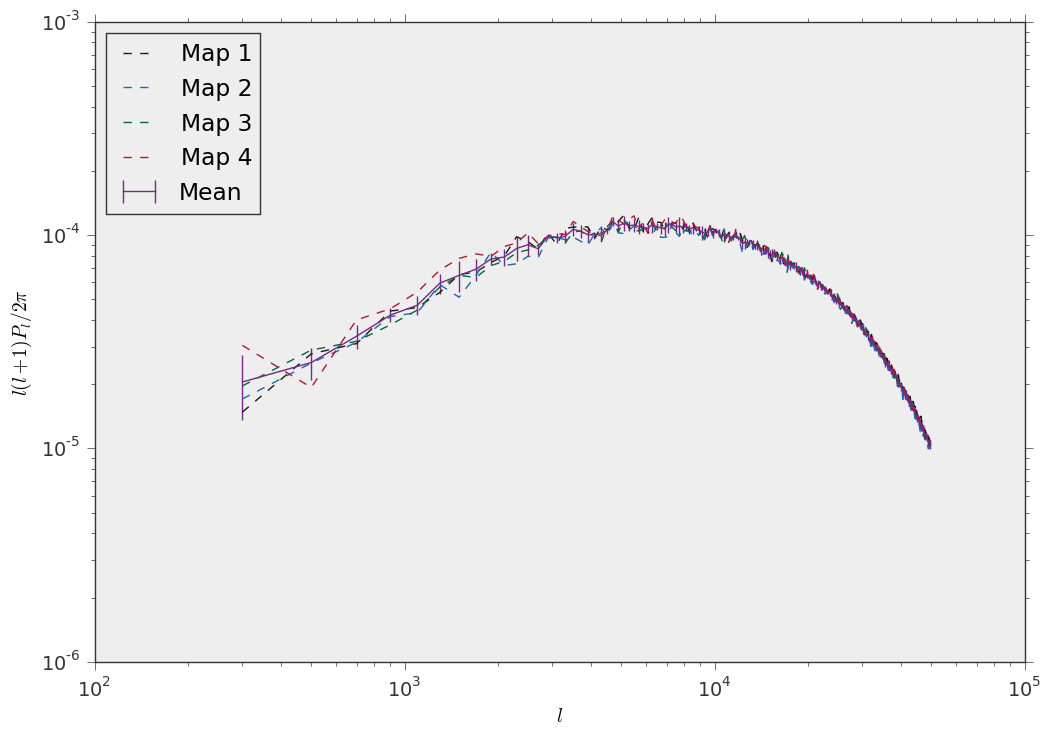# Measure the power spectrum of a convergence map (MPI)¶

When you need to measure the power spectrum of many different convergence maps (for example 1000 different realizations of a field of view), you are effectively measuring an Ensemble of quantities. lenstools provides Ensemble computing tools through the Ensemble class. This class supports parallel operations too through MPI, like in this example. First define a function that measures the power spectrum out of a single map:

#The operations on convergence maps are handled with the ConvergenceMap class
from lenstools import ConvergenceMap

def measure_power_spectrum(filename,l_edges):

l,Pl = conv_map.powerSpectrum(l_edges)
return Pl


This is the actual code:

from lenstools.statistics.ensemble import Ensemble
from lenstools.utils.decorators import Parallelize

import logging

import numpy as np
import matplotlib.pyplot as plt

logging.basicConfig(level=logging.DEBUG)

@Parallelize.masterworker
def main(pool):

l_edges = np.arange(200.0,50000.0,200.0)
l = 0.5*(l_edges[:-1] + l_edges[1:])

fig,ax = plt.subplots()
for n in range(len(conv_ensemble)):
ax.plot(l,l*(l+1)*conv_ensemble.iloc[n]/(2.0*np.pi),label="Map {0}".format(n+1),linestyle="--")

mean = conv_ensemble.mean(0)
errors = np.sqrt(conv_ensemble.covariance().values.diagonal())

ax.errorbar(l,l*(l+1)*mean/(2.0*np.pi),yerr=l*(l+1)*errors/(2.0*np.pi),label="Mean")

ax.set_xscale("log")
ax.set_yscale("log")
ax.set_xlabel(r"$l$")
ax.set_ylabel(r"$l(l+1)P_l/2\pi$")
ax.legend(loc="upper left")

plt.savefig("power_ensemble.png")

if __name__=="__main__":
main(None)


And this is the result